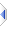# Example Program

The following is an example of a complete Phix program. For lots of other things you can run, try "pw pdemo" (or "./p pdemo" on linux).

```function merge_sort(sequence x)
-- put x into ascending order using a recursive merge sort
integer midpoint
sequence merged, first_half, second_half
if length(x)<=1 then
return x  -- trivial case
end if
midpoint = floor(length(x)/2)
first_half = merge_sort(x[1..midpoint])
second_half = merge_sort(x[midpoint+1..\$])
-- merge the two sorted halves into one
merged = {}
while length(first_half)>0
and length(second_half)>0 do
if first_half<=second_half then
merged = append(merged, first_half)
first_half = first_half[2..\$]
else
merged = append(merged, second_half)
second_half = second_half[2..\$]
end if
end while
-- result is the merged data plus any leftovers
return merged & first_half & second_half
end function

sequence list = {9, 10, 3, 1, 4, 5, 8, 7, 6, 2}
? merge_sort(list)
```
The above example delares a function, a sequence, and then invokes the function and displays the results. It also demonstrates how sequences (flexible arrays) are the real powerhouse of Phix.

The output from the program is:
```    {1, 2, 3, 4, 5, 6, 7, 8, 9, 10}
```
merge_sort() will just as easily sort {1.5, -9, 1e6, 100} or {"oranges", "apples", "bananas"}.

This example is stored as demo\mergesort.exw. The standard Phix distribution also contains nearly 500 other sample programs, including pdemo.exw which lists them all (and shows a count) with a simple filter/search mechanism, and can run or edit any that interest you.

Note that you would normally just use the builtin sort rather than write your own routine. If you need to sort huge amounts of data and would like to investigate alternative algorithms to find which works best with that data, see demo\allsorts.exw which contains nine of them along with testing/timing code to show performance with increasing amounts of data. The example demo\filesort.exw demonstrates practical use of the generic builtin sort routine.

In fact, the following single line of code is also a complete Phix program and gets exactly the same results as above

```    ? sort({9, 10, 3, 1, 4, 5, 8, 7, 6, 2})
```
Compatibility note: Euphoria requires "compare(first_half,second_half)<=0" in place of "first_half<=second_half" in the first example, and "include sort.e" in the second; the resulting source code also works on Phix. Obviously any further examples in this document are likewise written as clearly and succinctly as possible, rather than to maximise compatibility.